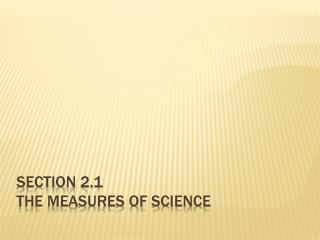DownloadDownload PresentationSection 2.1 The Measures of Science

# Section 2.1 The Measures of Science

Télécharger la présentation## Section 2.1 The Measures of Science

- - - - - - - - - - - - - - - - - - - - - - - - - - - E N D - - - - - - - - - - - - - - - - - - - - - - - - - - -
##### Presentation Transcript

1. Section 2.1The Measures of Science

2. Journal Entry #1 Develop and write a plan to determine the answer to the following question… How many drops of water will it take to fill the bucket? Materials: Colored water, eye dropper, graduated cylinder, meter stick 2.1 The Measures of Science

3. Objectives Define the SI standards of measurement Use common metric prefixes Estimate measurements and solutions to problems Perform arithmetic operations using scientific notation 2.1 The Measures of Science

4. We gotta have standards!!! 2.1 The Measures of Science

5. The Metric System and SI The Metric System was adopted by French scientists in 1795. Prior to the adoption of the Metric System, units of measurement were not standardized, but based on local customs. The Metric System provided a standard system that was in an easy to use, base ten system. 2.1 The Measures of Science

6. The system currently in use by most of the scientific community is an adaptation of the Metric System called SI (SystemeInternationaled’Unites). SI is regulated by an international organization. The standards of length, time, and mass are kept in France and Maryland. 2.1 The Measures of Science

7. Countries not yet using metric system…. US, Liberia, Myanmar 2.1 The Measures of Science

8. SI Base Units 2.1 The Measures of Science

9. The base units are defined based on some fixed standards. SI also recognizes derived units, which are combinations of the base units (ex. – m/s for speed, and kg*m2/s2) As you get more familiar with the derived units, you will be able to use something called dimensional analysis to help ensure the accuracy of your answers. 2.1 The Measures of Science

10. 2.1 The Measures of Science

11. Scientific notation is writing numbers as a number times a power of ten. Numbers in scientific notation are always in the form Mx10n where 1≤M<10 and n is an integer. To express a number in scientific notation, move the decimal point so there is one non-zero digit to the left of the decimal. Next, count the number of places you moved the decimal and that is the exponent on 10. 2.1 The Measures of Science

12. If you moved the decimal left, n is positive. If you moved it right, n is negative. Practice problems 1-3, p. 20 2.1 The Measures of Science

13. Factor-Label method (unit conversion) Remember that you can multiply 1 times anything without changing its value. A conversion factor is a multiplier equal to 1. Because 1kg = 1000g, the following factors can be established…1 = 1kg/1000g = 1000g/1kg Choose the conversion factor that cancels units in the way that is helpful to your problem. 2.1 The Measures of Science

14. Units cancel just like in algebraic equations. Sometimes, you may have to use more than one conversion factor if there is no direct conversion between units. Practice problems 4 & 5, p. 21 2.1 The Measures of Science

15. Assignment: p. 38-39, #’s 1-6, 30, 31 Key Terms for section 2.1 from p. 37 into your notebook. 2.1 The Measures of Science

16. Addition or Subtraction of numbers in scientific notation… Make sure numbers have the same exponent (convert one or the other, if necessary). Add or subtract the values of M and keep the same n. Be sure that your final answer is in proper scientific notation. Practice Problems 6-8, p. 22 2.1 The Measures of Science

17. Multiplication or Division of numbers in scientific notation… To multiply… multiply the M’s and add the n’s To divide… divide the M’s and subtract the n’s Be sure that your final answer is in proper scientific notation. Practice Problems 9-14, p. 23 2.1 The Measures of Science

18. Assignment: Physics Skills Packet 1, #’s 1-16 2.1 The Measures of Science